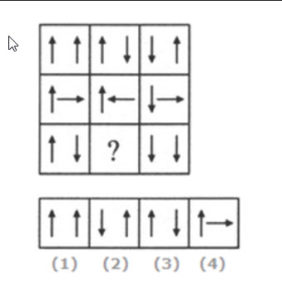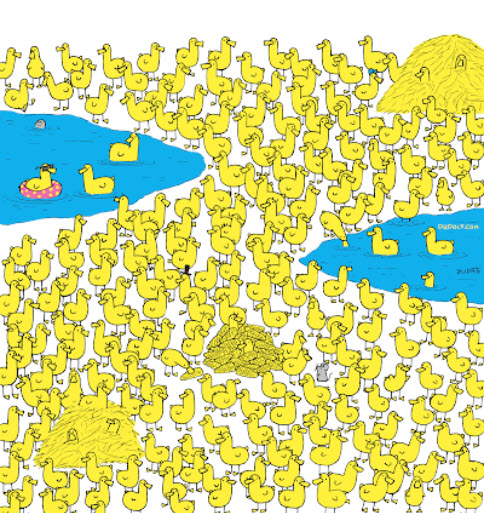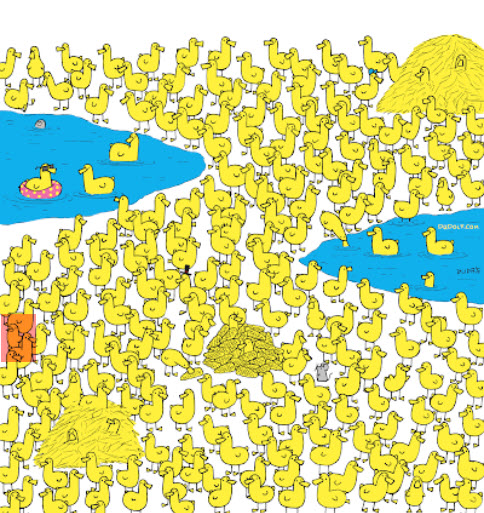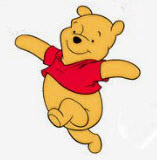Select Page

September 2022

##Option 1 completes the pattern

Explanation:

In each row, the second figure is obtained from the first figure by reversing the direction of the Right Hand Side arrow and the third figure is obtained from the second figure by reversing the direction of both the arrows.

##Did you spot the Chick – it was a challenge, I know!

The chick has a tiny pointed beak .

## Complete the Star

This WAS a challenge – of logic, trial and error and elimination.
Did you find the ‘trick’?
Multiply the same-colour sections and the answer always = 200
(* means multiply)
Blue – 50*4=200; Red 10*20=200; Grey 5*40=200; Pink 25*8=200; Orange 2*100=200

Green 1*200 =200

## D  L  B  N  A

Look out for some exciting news about future brain challenges – you’ll be one of the first to know!